# Adding a horizontal Benchmark/Baseline/Target Line to a Chart or Graph

This post will teach you how to add a horizontal benchmark line to an existing Excel chart or graph in excel. How do I add a horizontal baseline or target line to a chart or graph gives loads of context for the viewer.

For example, you have a simple Excel spreadsheet tracking product sales. And you have a column with dates and another with sales. And you have made a simple chart with both columns as data sources. And you want to add a horizontal baseline to this chart so that the user can see how close they are to their target sales. You can follow this guide to achieve the result.

## Adding a horizontal Benchmark/Baseline/Target Line to a Chart or Graph

To add a horizontal benchmark line to a chart, just do the following steps:

#1 create a new benchmark line column that contains benchmark sales data.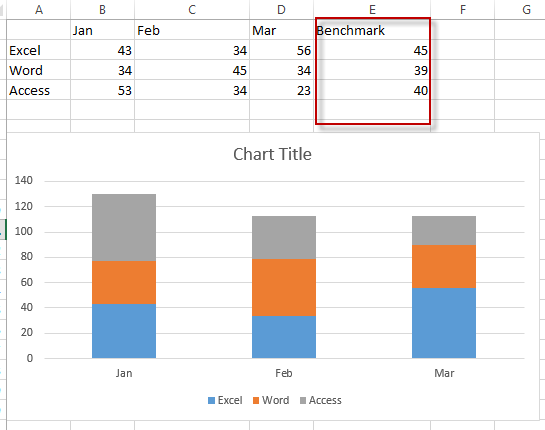#2 Right-click on the existing chart, and choose Select Data… from the drop-down menu list.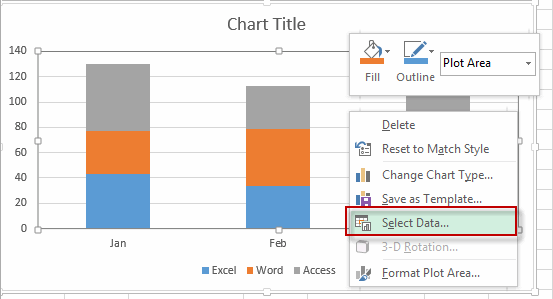#3 the Select Data source window will appear.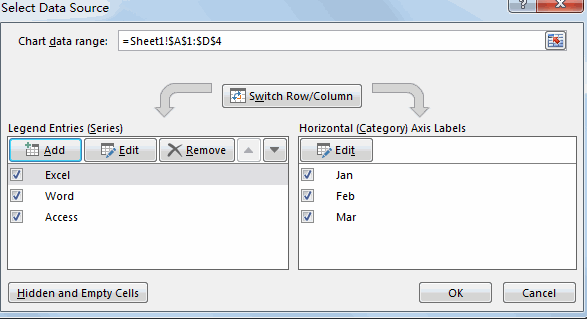#4 click Add button under Legend Entries section, the Edit Series window will appear.

#5 enter one Series name such as: Benchmark line into the Series name text box. Then select benchmark line column as its series values. Then click OK button.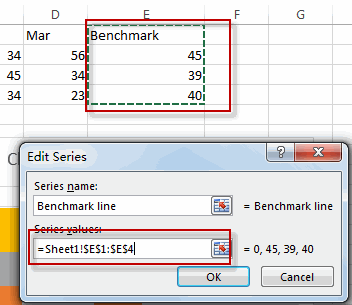#6 you will see that the benchmark line column has been added into the cart.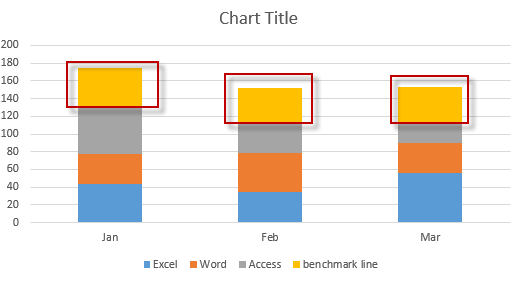#7 Right-click on the newly benchmark line series, and select Change Series Chart Type from the drop down menu list. The Change Chart Type window will appear.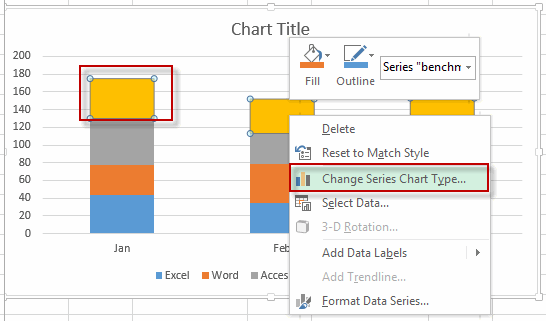#8 Select chart type as line for the benchmark line series.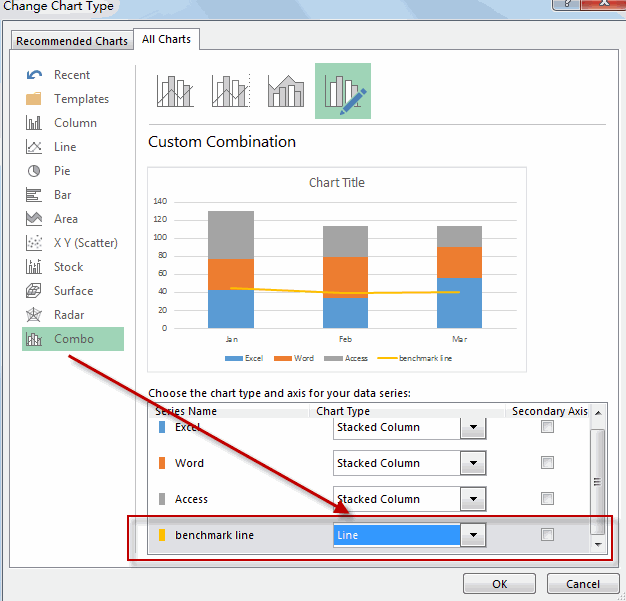So far, the horizontal benchmark line or baseline is added into the existing chart.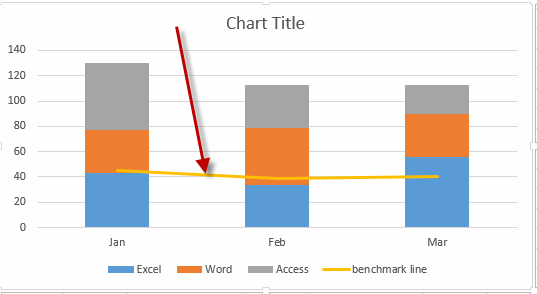Related Posts

Cap percentage values between 0 and 100

This article will talk about how to cap the percentage values between 0% and 100% in Microsoft Excel Spreadsheet or Google Sheets. If you are a newbie on Excel or google Sheets, you may be able to do this by ...

Calculate Cap Percentages to Specific Value

This article will talk about how to limit the cap percentage of a given amount to a given value in Microsoft Excel Spreadsheet or Google Sheets. If you are a newbie on Excel or google Sheets, you may be able ...

Calculate Win Loss Tie

Suppose you got a task to calculate the win, loss, and tie totals; what would you do? If you are new to Ms Excel and don't have enough experience with it, then you might do this task manually but let ...

Calculate Years Between Dates In Ms Excel

If you are an avid Ms Excel user, then you might have come across a task in which you needed to calculate the years between the dates; you might take it easy and do this task manually, which is also ...

Calculate Number of Hours between Two Times

Calculating the difference between two times might be a valuable statistic for subsequent computations or averages, whether you're producing a time sheet for staff or recording personal exercises. While Excel has a plethora of complex functions, including date and time ...

Calculate Loan Interest in Given Year

When you borrow money, you are supposed to repay it gradually. Lenders, on the other hand, want to be compensated for their services and the risk they incur by lending you money. That is, you will not just repay the ...

Calculate Interest Rate for Loan

The interest rate is the fee charged by a lender to a borrower and is expressed as a percentage of the principal—the lent amount. The interest rate on a loan is often expressed as an annual percentage rate, abbreviated as ...

Calculate Interest for Given Period

Using the IPMT function in Excel, we can compute the interest payment on any loan. This step-by-step tutorial will guide Excel users of all skill levels through the process to calculate interest for given period. Finally, the formula: =IPMT(B3/12,1,B5,-B2) The ...

How To Use Excel GCD Function

This post will guide you how to use Excel GCD function with syntax and examples in Microsoft excel. Description The Excel GCD function Returns the greatest common divisor of two or more integers. So you can use the GCD function ...

Calculate A Ratio From Two Numbers In Excel

In elementary mathematics, a ratio is a connection or comparison between two or more integers. For example, ratios are often expressed as ":" to demonstrate the relationship between two numbers. You would think that manually calculating a ratio from two ...

Sidebar